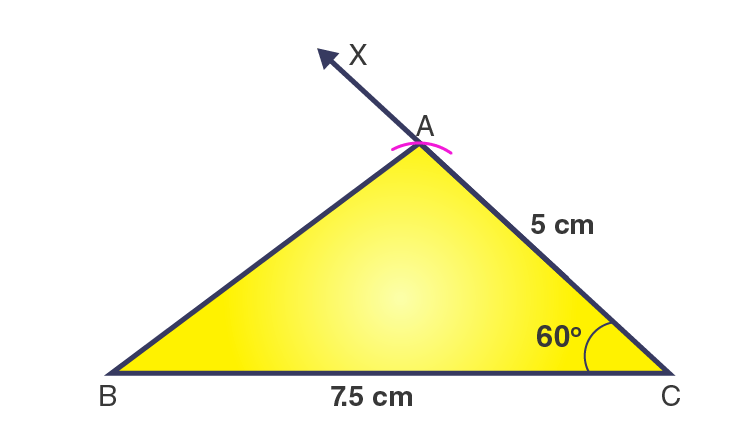# Construct ΔABC with BC = 7.5 cm, AC = 5 cm and m∠C = 60°.

SolutionThe steps for construction are given below:

1. Draw a line segment BC = 7.5 cm

2. At point C, draw a ray CX to making an angle of 60o i.e. ∠XCB = 60o.

3. Along CX, set off AC = 5cm.

4. Join AB.

Then, ΔABC is the required triangle.(0)(0)